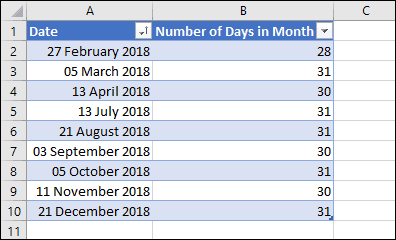# Excel formula to get number of days in a month

Sometimes you may need to extract the number of days in the month that a given date falls into, most likely when working with due dates or expiry dates.

This can be done using Excel’s DATE function, and can be done most easily by making use of some ‘secret’ knowledge of how the function works.

If you’re unfamiliar with Excel formulas and functions you could benefit greatly from our free Basic Skills E-book.
You can also find a much more in-depth look at the DATE function in our Expert Skills Books and E-books.

## The DATE function and day zero

It’s a little-known fact that Excel’s DATE function understands the concept of months having a ‘day zero’. Day zero is always the last day of the previous month.

Knowing this makes it easy to find out the number of days in the month for any date. All you need to do is find ‘day zero’ of the following month.

Here’s the formula, assuming your date is in cell A2:

=DAY(DATE(YEAR(A2),MONTH(A2)+1,0))

Assuming your date is the 5th of November 2018, this is equivalent to:

=DAY(DATE(2018,11+1,0)

## Sample workbook